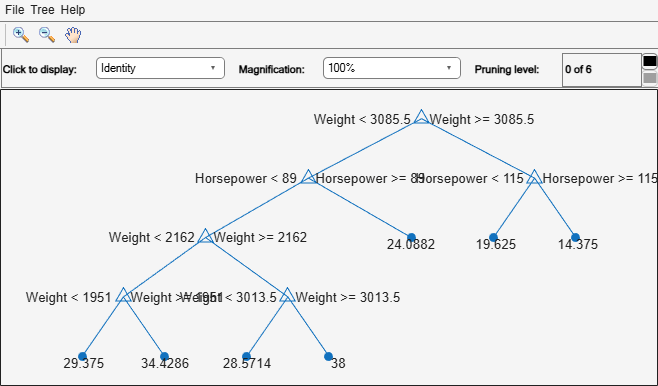# prune

Class: RegressionTree

Produce sequence of regression subtrees by pruning

## Syntax

```tree1 = prune(tree) tree1 = prune(tree,Name,Value) ```

## Description

`tree1 = prune(tree)` creates a copy of the regression tree `tree` with its optimal pruning sequence filled in.

`tree1 = prune(tree,Name,Value)` creates a pruned tree with additional options specified by one `Name,Value` pair argument. You can specify several name-value pair arguments in any order as `Name1,Value1,…,NameN,ValueN`.

## Input Arguments

 `tree` A regression tree created with `fitrtree`.

### Name-Value Arguments

Optional comma-separated pair of `Name,Value` arguments, where `Name` is the argument name and `Value` is the corresponding value. `Name` must appear inside single quotes (`''`). You can specify only one name-value pair argument.

 `Alpha` A numeric scalar from `0` (no pruning) to `1` (prune to one node). Prunes to minimize the sum of (`Alpha` times the number of leaf nodes) and a cost (mean squared error). `Level` A numeric scalar from `0` (no pruning) to the largest pruning level of this tree `max(tree.PruneList)`. `prune` returns the tree pruned to this level. `Nodes` A numeric vector with elements from `1` to `tree.NumNodes`. Any `tree` branch nodes listed in `Nodes` become leaf nodes in `tree1`, unless their parent nodes are also pruned.

## Output Arguments

 `tree1` A regression tree.

## Examples

expand all

Load the `carsmall` data set. Consider `Horsepower` and `Weight` as predictor variables.

```load carsmall; X = [Weight Horsepower]; varNames = {'Weight' 'Horsepower'};```

Grow a regression tree using the entire data set. View the tree.

`Mdl = fitrtree(X,MPG,'PredictorNames',varNames)`
```Mdl = RegressionTree PredictorNames: {'Weight' 'Horsepower'} ResponseName: 'Y' CategoricalPredictors: [] ResponseTransform: 'none' NumObservations: 94 Properties, Methods ```
`view(Mdl,'Mode','graph');`The regression tree has 16 pruning levels.

Prune the regression tree to pruning-level 10. View the pruned tree.

```MdlPruned = prune(Mdl,'Level',10); view(MdlPruned,'Mode','graph');```The pruned tree has six pruning levels.

Alternatively, you can use the pruning-level field in the Regression tree viewer to prune the tree.

## Tips

• `tree1 = prune(tree)` returns the decision tree `tree1` that is the full, unpruned `tree`, but with optimal pruning information added. This is useful only if you created `tree` by pruning another tree, or by using `fitrtree` with pruning set `'off'`. If you plan to prune a tree multiple times along the optimal pruning sequence, it is more efficient to create the optimal pruning sequence first.